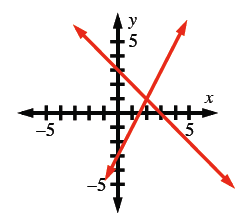### Home > AC > Chapter 4 > Lesson 4.2.3 > Problem4-92

4-92.

Graph the lines $y = 2x − 3$ and $y = −x + 3$.

Identify two points on each line. Graph those points.1. Where do they intersect? Label the point on the graph.

Remember that intersect means to cross.

1. Find the point of intersection using the Equal Values Method. That is, start by combining both equations into one equation that you can solve for $x$.

\begin{align*} y &= y \\ 2x − 3 &= −x + 3 \end{align*}

1. Which method is easier, graphing or using algebra to solve?